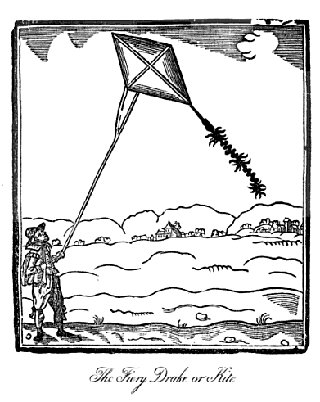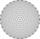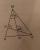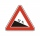# Kites

Boys run kite on a cable of 68 meters long. What is the kite altitude, if the angle from the horizontal plane is 72°?

Result

h =  65 m

#### Solution:

$l=68 \ \text{m} \ \\ \sin A=h:l \ \\ \ \\ h=l \cdot \ \sin( 72 ^\circ \rightarrow\ \text{rad} )=l \cdot \ \sin( 72 ^\circ \cdot \ \dfrac{ \pi }{ 180 } \ )=68 \cdot \ \sin( 72 ^\circ \cdot \ \dfrac{ 3.1415926 }{ 180 } \ )=64.67184=65 \ \text{m}$Our examples were largely sent or created by pupils and students themselves. Therefore, we would be pleased if you could send us any errors you found, spelling mistakes, or rephasing the example. Thank you!

Leave us a comment of this math problem and its solution (i.e. if it is still somewhat unclear...):Be the first to comment!Tips to related online calculators
Do you want to convert length units?

## Next similar math problems:

1. Cable carCable car rises at an angle 45° and connects the upper and lower station with an altitude difference of 744 m. How long is "endless" tow rope?
2. Cable car 2Cable car rises at an angle 41° and connects the upper and lower station with an altitude difference of 1175 m. How long is the track of cable car?
3. The cable carThe cable car has a length of 3,5 kilometers and an angle of climb of 30 degrees. What is the altitude difference between Upper and Lower Station?
4. 30-60-90The longer leg of a 30°-60°-90° triangle measures 5. What is the length of the shorter leg?
5. Internal and external anglesCalculate the remaining internal and external angles of a triangle, if you know the internal angle γ (gamma) = 34 degrees and one external angle is 78 degrees and 40 '. Determine what kind of triangle it is from the size of its angles.
6. Outer anglesThe outer angle of the triangle ABC at the A vertex is 71°40 ' outer angle at the vertx B is 136°50'. What size has the inner triangle angle at the vertex C?
7. N-gon anglesWhat is the sum of interior angles 8-gon? What is the internal angle of a regular convex 8-polygon?
8. Obtuse angleThe line OH is the height of the triangle DOM, line MN is the bisector of angle DMO. obtuse angle between the lines MN and OH is four times larger than the angle DMN. What size is the angle DMO? (see attached image)
9. Hexagon 5The distance of parallel sides of regular hexagonal is 61 cm. Calculate the length of the radius of the circle described to this hexagon.
10. An angleAn angle x is opposite side AB which is 10, and side AC is 15 which is hypotenuse side in triangle ABC. Calculate angle x.
11. Angles of a triangleIn the triangle ABC, the angle beta is 15° greater than the angle alpha. The remaining angle is 30° greater than the sum of the angles alpha and beta. Calculate the angles of a triangle.
12. Right angleIf a, b and c are two sides of a triangle ABC, a right angle in A, find the value on each missing side. If b=10, c=6
13. Uphill gardenI have a garden uphill, increasing from 0 to 4.5 m for a length of 25 m, how much is the climb in percent?
14. Height 2Calculate the height of the equilateral triangle with side 38.
15. Center traverseIt is true that the middle traverse bisects the triangle?The ladder has a length of 3 m and is leaning against the wall, and its inclination to the wall is 45°. How high does it reach?Stairway has 20 steps. Each step has a length of 22 cm and a height of 15 cm. Calculate the length of the handrail of staircases if on the top and bottom exceeds 10 cm.